# OECD Tourism flows data analysis 4 - Time series regression for Japan tourism data with R.UnsplashHan Chenxuが撮影した写真

This post is floowing of the above post.
In the above post, I find year 2020 and 2021 have COVID-19 effect and each locations has their own characteristics.

So I will focus a few locations without 2020 and 2021.

Since I live in Japan, let's focus on JPN.

I made a data frame which contains JPN only and without 2020 and 2021.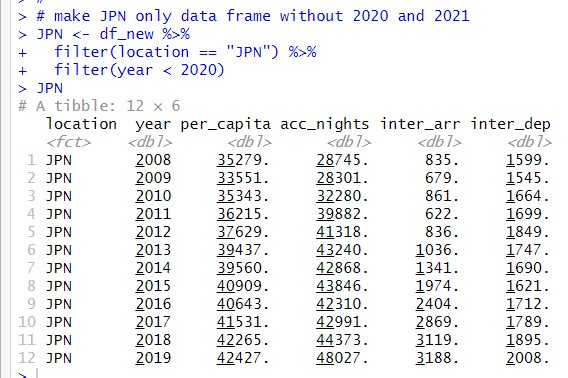Let's see per_capita, acc_nights, inter_arr and inter_dep line grpah with geom_line() function.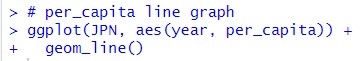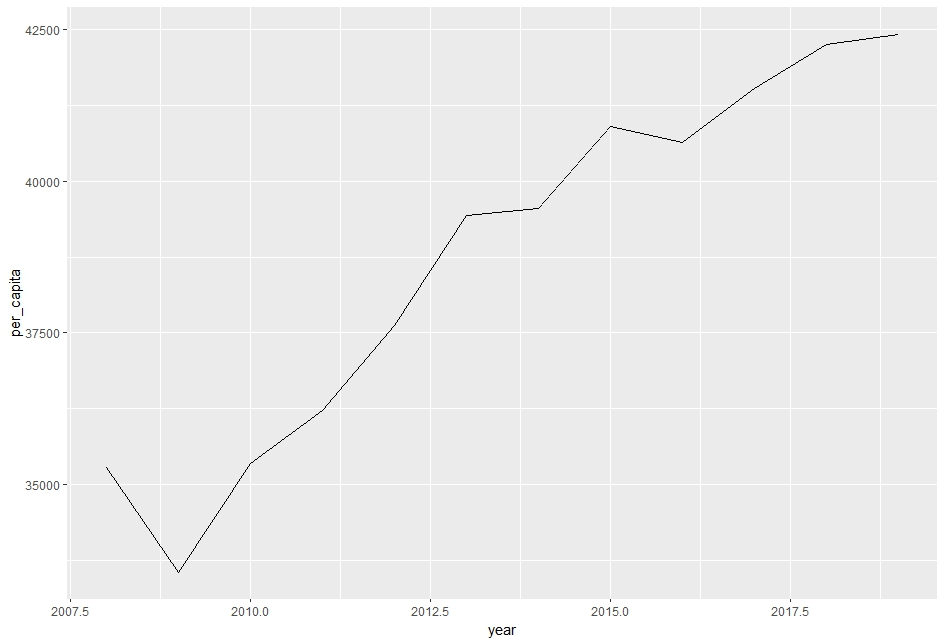per_capita has up trend.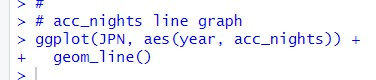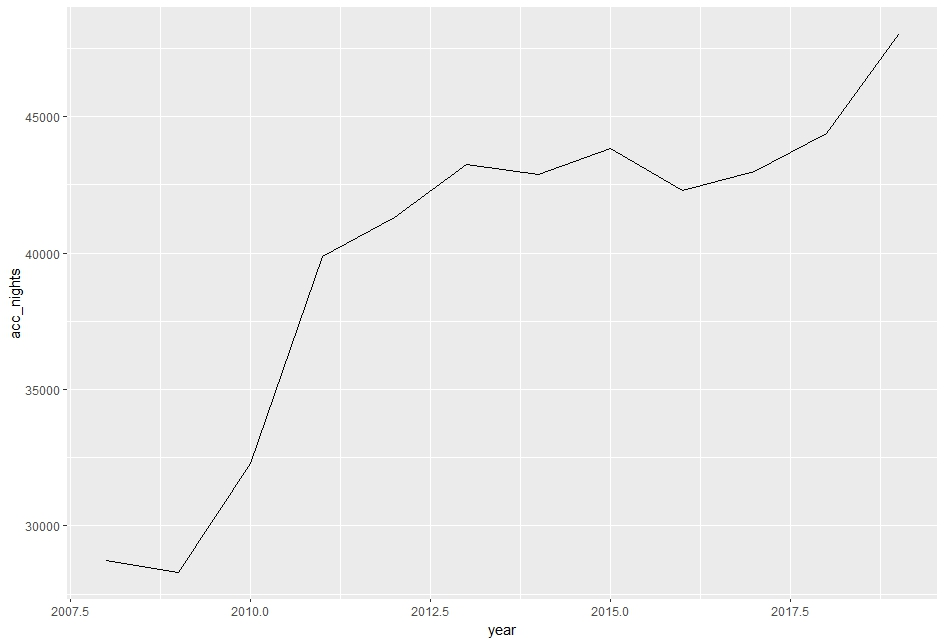acc_nights has up trend too.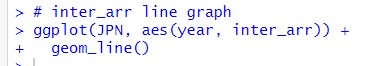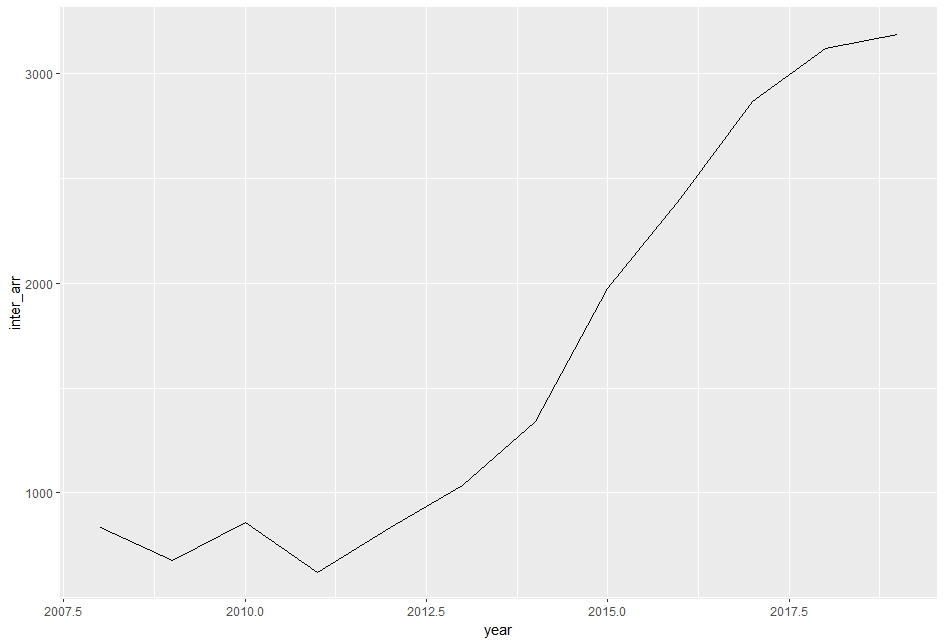inter_arr has up trend too.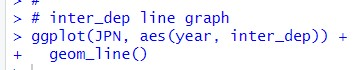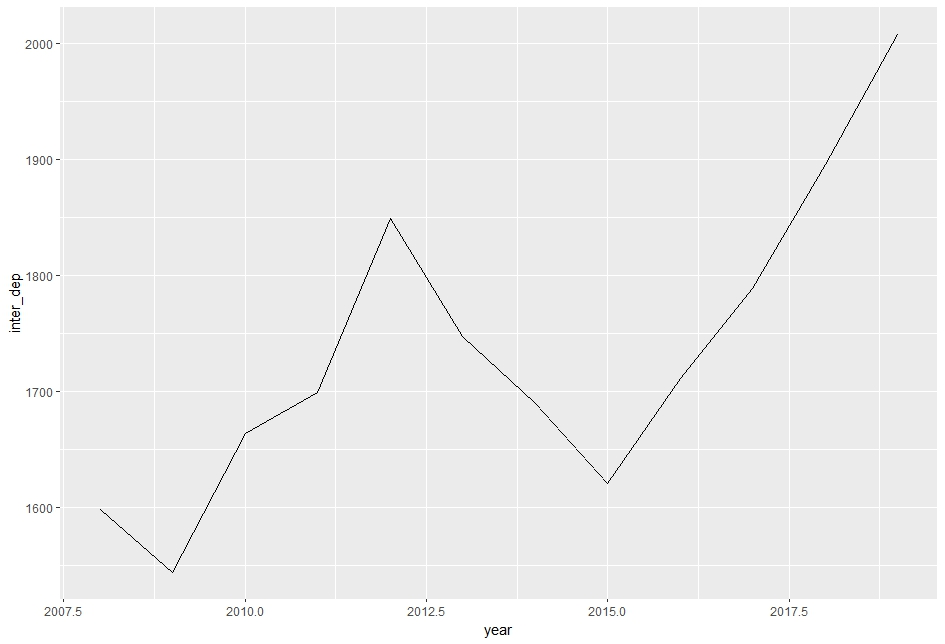inter_dep has up trend but it has double bottom in 2009 and 2025.

So, all four variables have clear up trend. Then, I will add time trend variable.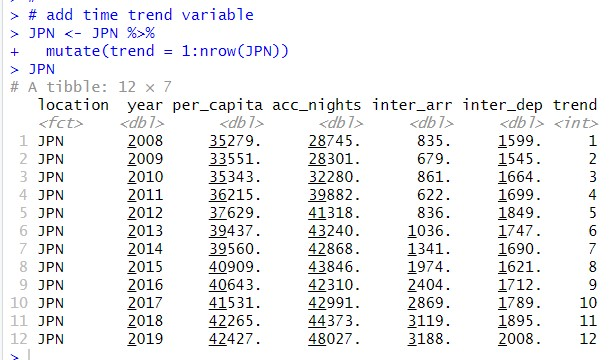I will do regression analysis for acc_nights, inter_arr and inter_dep on per_capita and trend.

First, let's do acc_nights.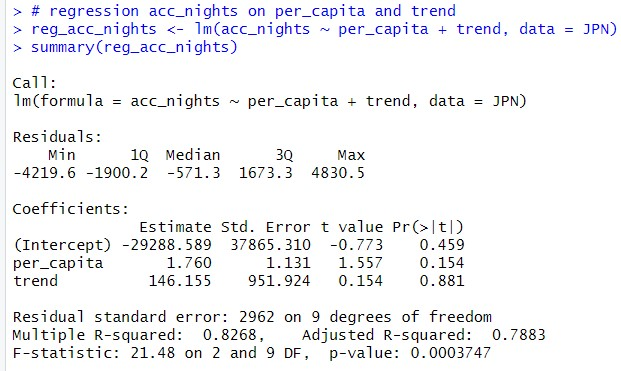p-value is less than 0.05 and this model seems valid. coefficient of per_capita is 1.760.

So, when per_capita increase by 1, acc_nights will increase 1.760. but p-value is 0.154 so, I cannot say per_capita is statistically significant.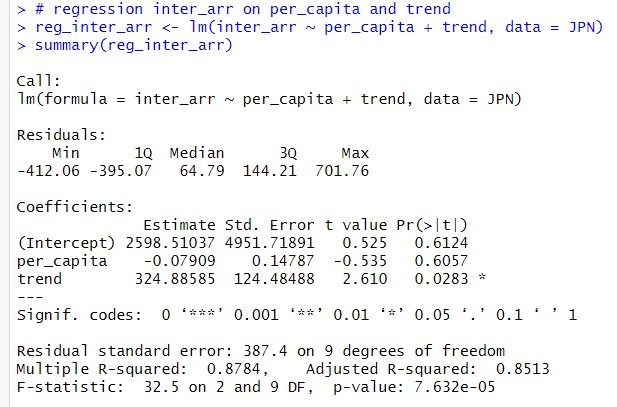p-value is less than 0.05. So this model is not invalid. The coefficient of per_capita is -0.07909 and p-value is 0.6057. I can say per_capita is not significant for inter_arr.

The coefficient of trend is 324.88585 and p-value is 0.0283. So trend is statistically sgnificant. Each year increase 324 inter_arr.

Last, let's see inter_dep.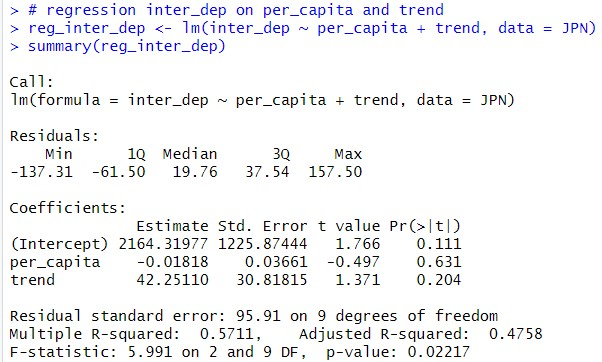p-value is less than 0.05(0.02217) but per_capita p-value is 0.631. So I cannot say per_capita is statistically significant.

That's it. Thank you!

Next post is,

To read from the 1st post,